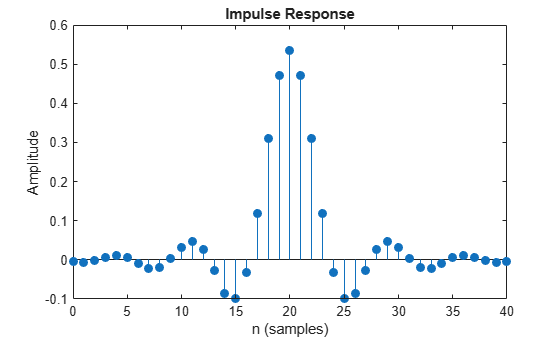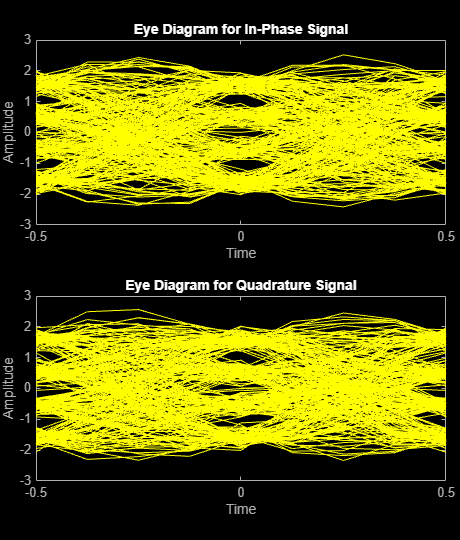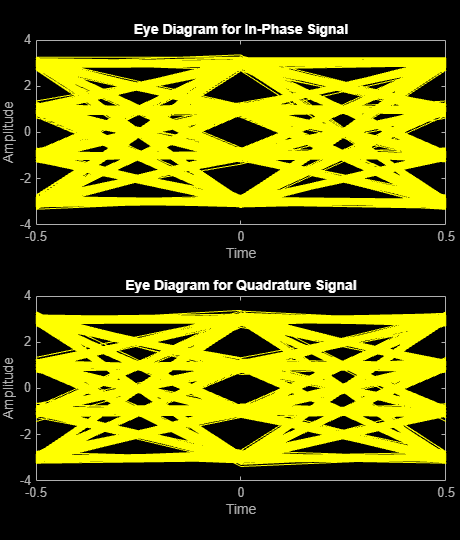# Use Pulse Shaping on 16-QAM Signal

This example extends the Examine 16-QAM Using MATLAB example to perform pulse shaping and raised cosine filtering by using a pair of square-root raised cosine (RRC) filters. The `rcosdesign` function creates the filters. BER performance can be improved by adding forward error correction (FEC) to the communication link. To add FEC to the communications link with pulse shape filtering example, see the Use Forward Error Correction on 16-QAM Signal example.

This example shows how to process a binary data stream by using a communications link that consists of a baseband modulator, channel, demodulator, and pulse shaping and raised cosine filtering. The example computes the bit error rate (BER), displays filter effects in eye diagrams, and displays the transmitted and received signals in a constellation diagram.

### Establish Simulation Framework

Define simulation parameters for a 16-QAM modulation scheme with raised cosine filtering, and an AWGN channel.

```M = 16; % Modulation order k = log2(M); % Bits per symbol numBits = k*7.5e4; % Bits to process sps = 4; % Samples per symbol (oversampling factor)```

#### Create RRC Filter

Set the RRC filter parameters.

```filtlen = 10; % Filter length in symbols rolloff = 0.25; % Filter rolloff factor```

Use the `rcosdesign` function to create an RRC filter.

`rrcFilter = rcosdesign(rolloff,filtlen,sps);`

Use the FVTool to display the RRC filter impulse response.

`fvtool(rrcFilter,'Analysis','Impulse')`### Compute System BER

Use the `randi` function to generate random binary data. Set the `rng` function to its default state, or any static seed value, so that the example produces repeatable results.

```rng default; % Use default random number generator dataIn = randi([0 1],numBits,1); % Generate vector of binary data```

Use the `bit2int` function to convert `k`-tuple binary words into integer symbols.

`dataSymbolsIn = bit2int(dataIn,k);`

Apply 16-QAM modulation using the `qammod` function.

`dataMod = qammod(dataSymbolsIn,M);`

Use the `upfirdn` function to upsample the signal by the oversampling factor and apply the RRC filter. The `upfirdn` function pads the upsampled signal with zeros at the end to flush the filter. Then, the function applies the filter.

`txFiltSignal = upfirdn(dataMod,rrcFilter,sps,1);`

Using the number of bits per symbol (`k`) and the number of samples per symbol (`sps`), convert the ratio of energy per bit to noise power spectral density (`EbNo`) to an SNR value for use by the `awgn` function.

```EbNo = 10; snr = EbNo + 10*log10(k) - 10*log10(sps);```

Pass the filtered signal through an AWGN channel.

`rxSignal = awgn(txFiltSignal,snr,'measured');`

Use the `upfirdn` function on the received signal to downsample and filter the signal. Downsample by using the same oversampling factor applied for upsampling the transmitted signal. Filter by using the same RRC filter applied to the transmitted signal.

Each filtering operation delays the signal by half of the filter length in symbols, `filtlen`/2. So, the total delay from transmit and receive filtering equals the filter length, `filtlen`. For the BER computation, the transmitted and received signals must be the same size and you must account for the delay between the transmitted and received signal. Remove the first `filtlen` symbols in the decimated signal to account for the cumulative delay of the transmit and receive filtering operations. Remove the last `filtlen` symbols in the decimated signal to ensure the number of samples in the demodulator output matches the number of samples in the modulator input.

```rxFiltSignal = ... upfirdn(rxSignal,rrcFilter,1,sps); % Downsample and filter rxFiltSignal = ... rxFiltSignal(filtlen + 1:end - filtlen); % Account for delay```

Use the `qamdemod` function to demodulate the received filtered signal.

`dataSymbolsOut = qamdemod(rxFiltSignal,M);`

Convert the recovered integer symbols into binary data by using the `int2bit` function.

`dataOut = int2bit(dataSymbolsOut,k);`

Determine the number of errors and the associated BER by using the `biterr` function.

```[numErrors,ber] = biterr(dataIn,dataOut); fprintf('\nFor an EbNo setting of %3.1f dB, the bit error rate is %5.2e, based on %d errors.\n', ... EbNo,ber,numErrors)```
```For an EbNo setting of 10.0 dB, the bit error rate is 1.79e-03, based on 538 errors. ```

### Visualize Filter Effects

To visualize the filter effects in an eye diagram, reduce the ${\mathit{E}}_{\mathrm{b}}/{\mathit{N}}_{0}$ setting and regenerate the received data. Visualizing a high SNR signal with no other multipath effects, you can use eye diagrams to highlight the intersymbol interference (ISI) reduction at the output for the pair of pulse shaping RRC filters. The RRC filter does not have zero-ISI until it is paired with the second RRC filter to form in cascade a raised cosine filter.

```EbNo = 20; snr = EbNo + 10*log10(k) - 10*log10(sps); rxSignal = awgn(txFiltSignal,snr,'measured'); rxFiltSignal = ... upfirdn(rxSignal,rrcFilter,1,sps); % Downsample and filter rxFiltSignal = ... rxFiltSignal(filtlen + 1:end - filtlen); % Account for delay```

Create an eye diagram for a portion of the filtered noiseless signal to visualize the effect of the pulse shaping. The transmitted signal has RRC filtering and shows ISI as a narrowing of the eye-opening.

`eyediagram(txFiltSignal(1:2000),sps*2);`Displaying the eye diagram of the signal after the channel noise shows the signal with RRC filtering and noise. The noise level causes further narrowing of the eye diagram eye-opening.

`eyediagram(rxSignal(1:2000),sps*2);`Displaying the eye diagram of the signal after the receive filtering is applied shows the signal with raised cosine filtering. The wider eye diagram eye-openings, the signal has less ISI with raised cosine filtering as compared to the signal with RRC filtering.

`eyediagram(rxFiltSignal(1:2000),2);`Create a constellation diagram of the received signal before and after filtering. Scale the received signal by the square root of the number of samples per symbol to normalize the transmit and receive power levels.

```scatplot = scatterplot(sqrt(sps)*... rxSignal(1:sps*5e3),... sps,0); hold on; scatterplot(rxFiltSignal(1:5e3),1,0,'bx',scatplot); title('Received Signal, Before and After Filtering'); legend('Before Filtering','After Filtering'); axis([-5 5 -5 5]); % Set axis ranges hold off;```